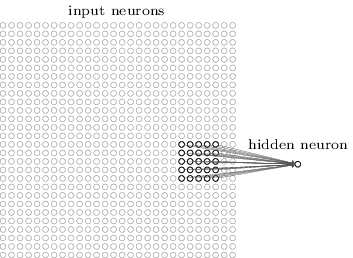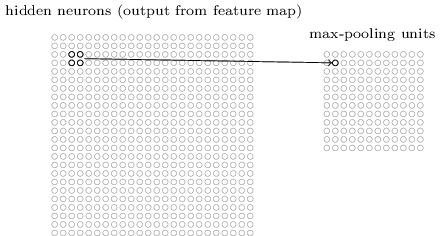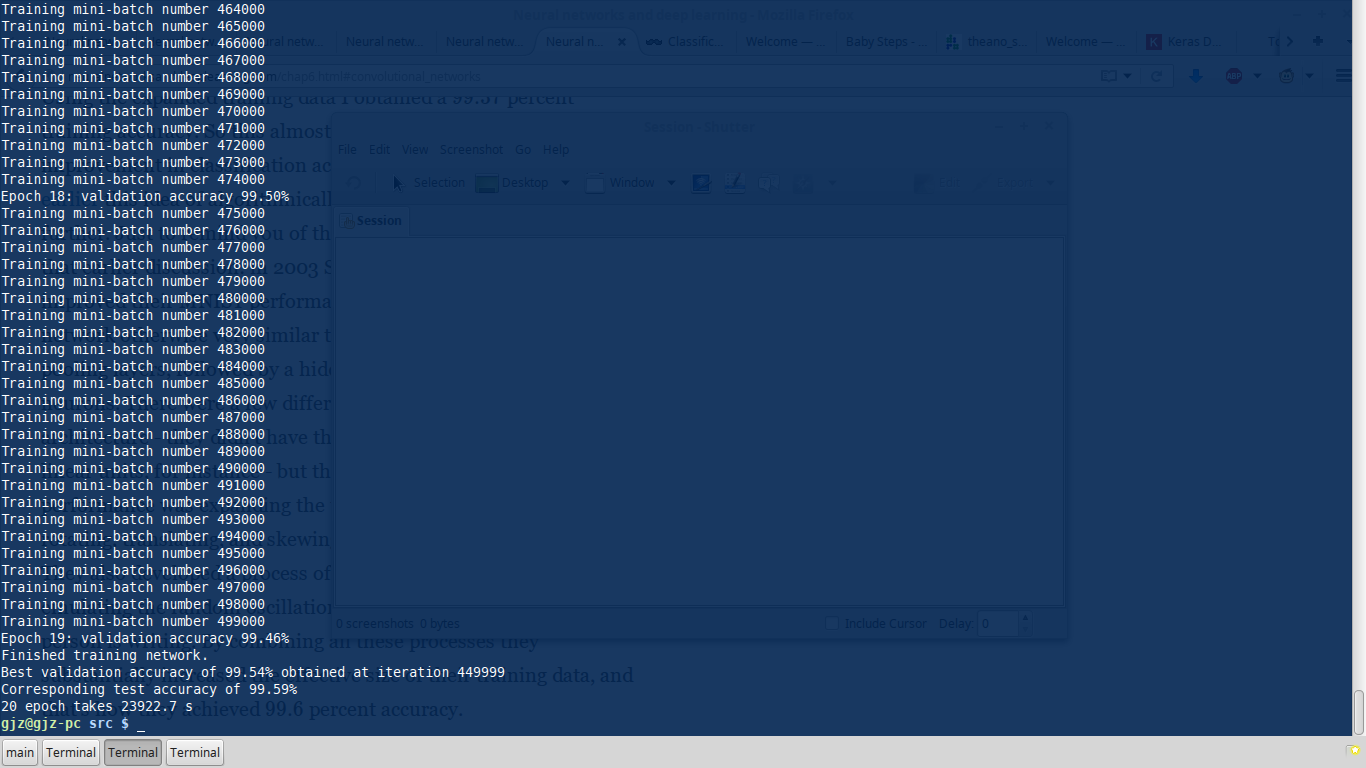@cleardusk 2015-11-27T11:39:23.000000Z 字数 2770 阅读 1453

# DL 在线书籍源码阅读（三）

GjzCVCode

## 2.2 CNN

This program incorporates ideas from the Theano documentation on
convolutional neural nets (notably,
http://deeplearning.net/tutorial/lenet.html ), from Misha Denil's
implementation of dropout (https://github.com/mdenil/dropout ), and
from Chris Olah (http://colah.github.io )self.

### 2.2.1 DNN

training_data, validation_data, test_data = \        mnist_loader.load_data_wrapper()    net = network2.Network([784, 30, 30, 30, 30, 10], cost=network2.CrossEntropyCost)    net.default_weight_initializer() # use another weight initialization method    net.SGD(training_data, 30, 10, 0.1, lmbda=5.0, evaluation_data=validation_data,            monitor_evaluation_accuracy=True)

• Instability to gradient-based learning(vanishing, exploding)
• The choice of activication function
• The way weights are initialized
• Details of how learning by gradieng descent is implemented

### 2.2.2 CNN

• Local receptive fields• Shared weights
比如上图中的 25 条 weights 是被共享的，所有这样的 weights 都是一样的。

σ(b+l=04m=04wl,maj+l,k+m).(3)

• Pooling layers### 2.2.3 一些 Tricks

• Using rectified linear units，就是 ReLu，caffe 的一个示例用的也是这个。
• Expanding the training data，这个前面也说过，这里将原始数据 expand 了五倍，往上下左右各移动一个像素加上原始的，是原数据的 5 倍。
• Using an ensemble of networks， 这个 trick 还没用，我现在也不清楚到底怎么用，到底如何将几个 network 的结果 ensemble，文中并没有给示例代码。

### 2.2.4 Run

def test_best():    """    One epoch takes 1196.6 s.    """    epoch = 20    training_data, validation_data, test_data = network3.load_data_shared()    expanded_training_data, _, _ = network3.load_data_shared(        "../data/mnist_expanded.pkl.gz")    mini_batch_size = 10    net = Network([        ConvPoolLayer(image_shape=(mini_batch_size, 1, 28, 28),                      filter_shape=(20, 1, 5, 5),                      poolsize=(2, 2),                      activation_fn=ReLU),        ConvPoolLayer(image_shape=(mini_batch_size, 20, 12, 12),                      filter_shape=(40, 20, 5, 5),                      poolsize=(2, 2),                      activation_fn=ReLU),        FullyConnectedLayer(n_in=40*4*4, n_out=1000,                            activation_fn=ReLU, p_dropout=0.5),        FullyConnectedLayer(n_in=1000, n_out=1000,                            activation_fn=ReLU, p_dropout=0.5),        SoftmaxLayer(n_in=1000, n_out=10, p_dropout=0.5)],        mini_batch_size)    time_begin = time.clock()    net.SGD(expanded_training_data, epoch, mini_batch_size, 0.03, validation_data,            test_data)    time_end = time.clock()    print '%d epoch takes %.1f s' % (epoch, (time_end - time_begin))• 私有
• 公开
• 删除